Next: Summary of problems and Up: Iterative methods Previous: Genetic programming

### Jacobian transpose method

Jacobian transpose method removes the problematic Jacobian inversion mentioned above. The annoying inversion is replaced by a simple transposition [Wel93]. The idea is based on the principle of virtual works and generalized forces [Pau81].

The external force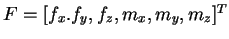(consisting of pull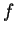and twist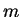) is applied to the end effector of the articulated structure and results internal forces and torques in the joints. The relation between the force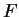and the generalized forces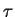is expressed as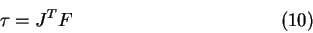The generalized forcescould be expressed using either the joint variable accelerations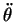or joint velocities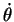[NN90]. The joint accelerations could be used for an accurate dynamic simulation of the manipulator motion. Because the method is not interested in the dynamic behavior, only the joint velocitiesare used for the necessity of this method. Thus equation (10) is supplied by another form: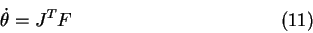The force is proportional to the velocity in equation (11). That means the object moves as long as the force takes effect. The inertia and torques are not applied. The scheme of the iterative model is demonstrated by the Figure 4.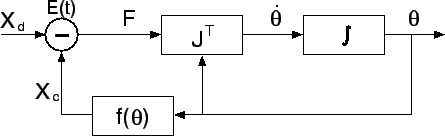The forcecorresponds to error function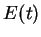expressed by equation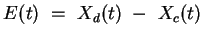.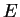is the error along the time-varying trajectory,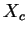is current position and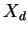is desired trajectory of the end effector.Next: Summary of problems and Up: Iterative methods Previous: Genetic programming
Lukas Barinka 2002-03-21